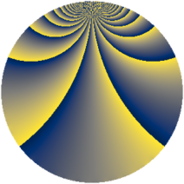# Properties

 Label 847.2.rLevel $847$ Weight $2$ Character orbit 847.r Rep. character $\chi_{847}(40,\cdot)$ Character field $\Q(\zeta_{30})$ Dimension $512$ Newform subspaces $6$ Sturm bound $176$ Trace bound $3$

# Related objects

## Defining parameters

 Level: $$N$$ $$=$$ $$847 = 7 \cdot 11^{2}$$ Weight: $$k$$ $$=$$ $$2$$ Character orbit: $$[\chi]$$ $$=$$ 847.r (of order $$30$$ and degree $$8$$) Character conductor: $$\operatorname{cond}(\chi)$$ $$=$$ $$77$$ Character field: $$\Q(\zeta_{30})$$ Newform subspaces: $$6$$ Sturm bound: $$176$$ Trace bound: $$3$$ Distinguishing $$T_p$$: $$2$$

## Dimensions

The following table gives the dimensions of various subspaces of $$M_{2}(847, [\chi])$$.

Total New Old
Modular forms 800 640 160
Cusp forms 608 512 96
Eisenstein series 192 128 64

## Trace form

 $$512q + 5q^{2} + 9q^{3} - 51q^{4} + 15q^{5} + 5q^{7} - 39q^{9} + O(q^{10})$$ $$512q + 5q^{2} + 9q^{3} - 51q^{4} + 15q^{5} + 5q^{7} - 39q^{9} - 108q^{12} + 16q^{14} + 71q^{16} - 15q^{17} - 20q^{18} + 15q^{19} - 50q^{23} - 75q^{24} - 31q^{25} + 33q^{26} + 40q^{28} + 40q^{29} - 25q^{30} - 9q^{31} - 5q^{35} + 18q^{36} - 3q^{37} - 63q^{38} + 45q^{39} - 75q^{40} - 16q^{42} - 36q^{45} + 20q^{46} - 9q^{47} - 65q^{49} - 30q^{50} - 55q^{51} + 15q^{52} + 5q^{53} - 96q^{56} - 60q^{57} - 62q^{58} + 27q^{59} + 67q^{60} + 30q^{61} + 40q^{63} - 20q^{64} - 108q^{67} + 75q^{68} + 47q^{70} - 36q^{71} + 60q^{72} + 60q^{73} - 45q^{74} + 33q^{75} - 364q^{78} + 70q^{79} + 99q^{80} + 11q^{81} + 153q^{82} + 125q^{84} - 10q^{85} + 50q^{86} - 102q^{89} - 60q^{91} + 30q^{92} + 64q^{93} - 105q^{94} - 30q^{95} - 75q^{96} + O(q^{100})$$

## Decomposition of $$S_{2}^{\mathrm{new}}(847, [\chi])$$ into newform subspaces

Label Dim. $$A$$ Field CM Traces $q$-expansion
$$a_2$$ $$a_3$$ $$a_5$$ $$a_7$$
847.2.r.a $$48$$ $$6.763$$ None $$-5$$ $$6$$ $$15$$ $$10$$
847.2.r.b $$48$$ $$6.763$$ None $$0$$ $$6$$ $$0$$ $$0$$
847.2.r.c $$48$$ $$6.763$$ None $$5$$ $$-9$$ $$-15$$ $$5$$
847.2.r.d $$48$$ $$6.763$$ None $$5$$ $$6$$ $$15$$ $$-10$$
847.2.r.e $$96$$ $$6.763$$ None $$0$$ $$0$$ $$0$$ $$0$$
847.2.r.f $$224$$ $$6.763$$ None $$0$$ $$0$$ $$0$$ $$0$$

## Decomposition of $$S_{2}^{\mathrm{old}}(847, [\chi])$$ into lower level spaces

$$S_{2}^{\mathrm{old}}(847, [\chi]) \cong$$ $$S_{2}^{\mathrm{new}}(77, [\chi])$$$$^{\oplus 2}$$